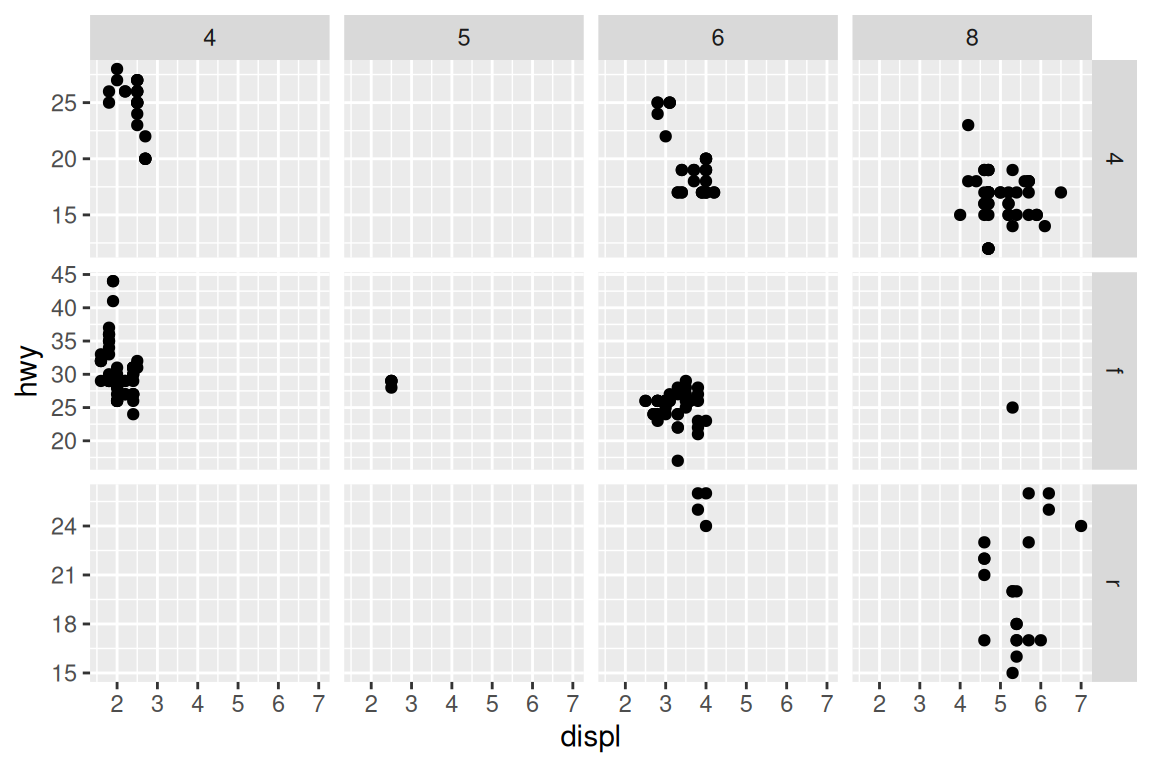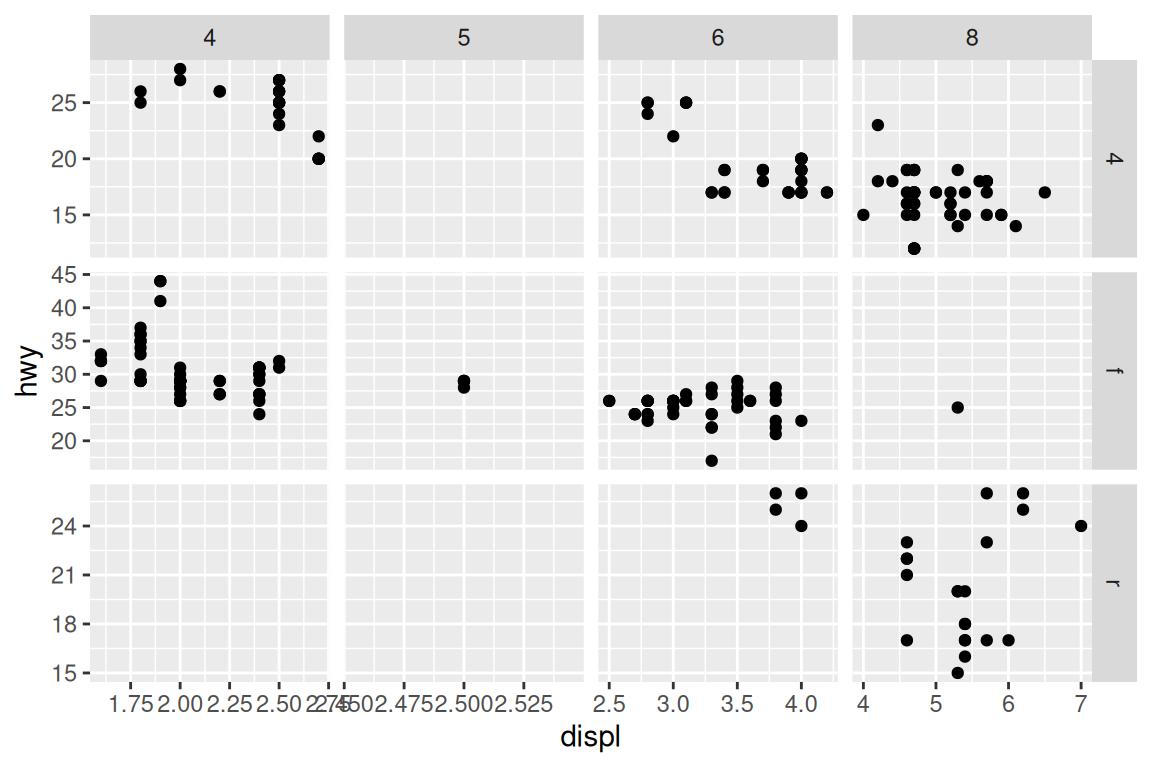## 11.2 Using Facets with Different Axes

### 11.2.1 Problem

You want subplots with different ranges or items on their axes.

### 11.2.2 Solution

Set the scales to `"free_x"`, `"free_y"`, or `"free"` (Figure 11.3):

``````# Create the base plot
mpg_plot <- ggplot(mpg, aes(x = displ, y = hwy)) +
geom_point()

# With free y scales
mpg_plot +
facet_grid(drv ~ cyl, scales = "free_y")

# With free x and y scales
mpg_plot +
facet_grid(drv ~ cyl, scales = "free")``````Figure 11.3: With free y scales (top); With free x and y scales (bottom)

### 11.2.3 Discussion

Each row of subplots has its own y range when free y scales are used; the same applies to columns when free x scales are used.

It’s not possible to directly set the range of each row or column, but you can control the ranges by dropping unwanted data (to reduce the ranges), or by adding `geom_blank()` (to expand the ranges).